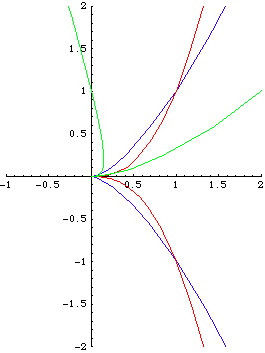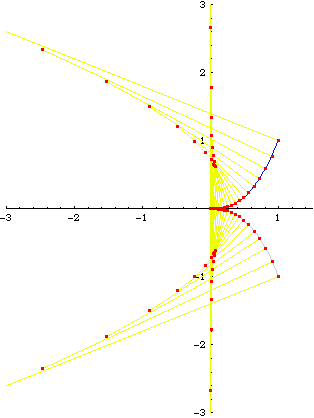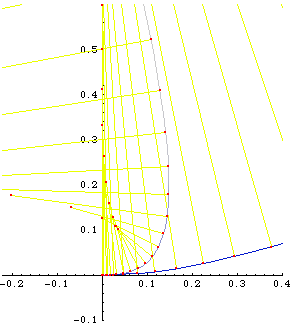# CuspsThree curves {t^2, t^3} (blue), {t^2, t^5} (red), {t^2 + t^3, t^4} (green). All have a cusp at t==0. The left image shows a plot, the right is a close up.

Their curvature functions are:

``` (6*t^2)/(t^2*(4 + 9*t^2))^(3/2)
(30*t^4)/(t^2*(4 + 25*t^6))^(3/2)
(-4*t^3*(2 + 6*t) + 12*t^2*(2*t + 3*t^2))/(16*t^6 + t^2*(2 + 3*t)^2)^(3/2)
```

Curvature at t=0 is undefined, but we can compute the limit at t=0. The following are plots of each curve and their Evolute. From these we learn that cusps can have a well-defined curvature, and may be different.{t^2, t^3} and its evolute. As one can see, as t approches the cusp, the radius of osculating circles (shown as yellow lines) decreases, and becomes 0 as a limit at the cusp. In other words, as t approaches the cusp, the curve gets more and more curved, and is infinitly curved like circle of radius 0 at the cusp.{t^2, t^5} and its evolute. The curve gets flatter and flatter near the cusp. The curvature at origin is 0. (as flat as a line).{t^2 + t^3, t^4} and its evolute. The curvature at origin is 2 (like the arc of a circle with radius 1/2).

cusps.nb

Example on this page are from C G Gibson's Elementary Geometry of Differential Curves Buy at amazon .